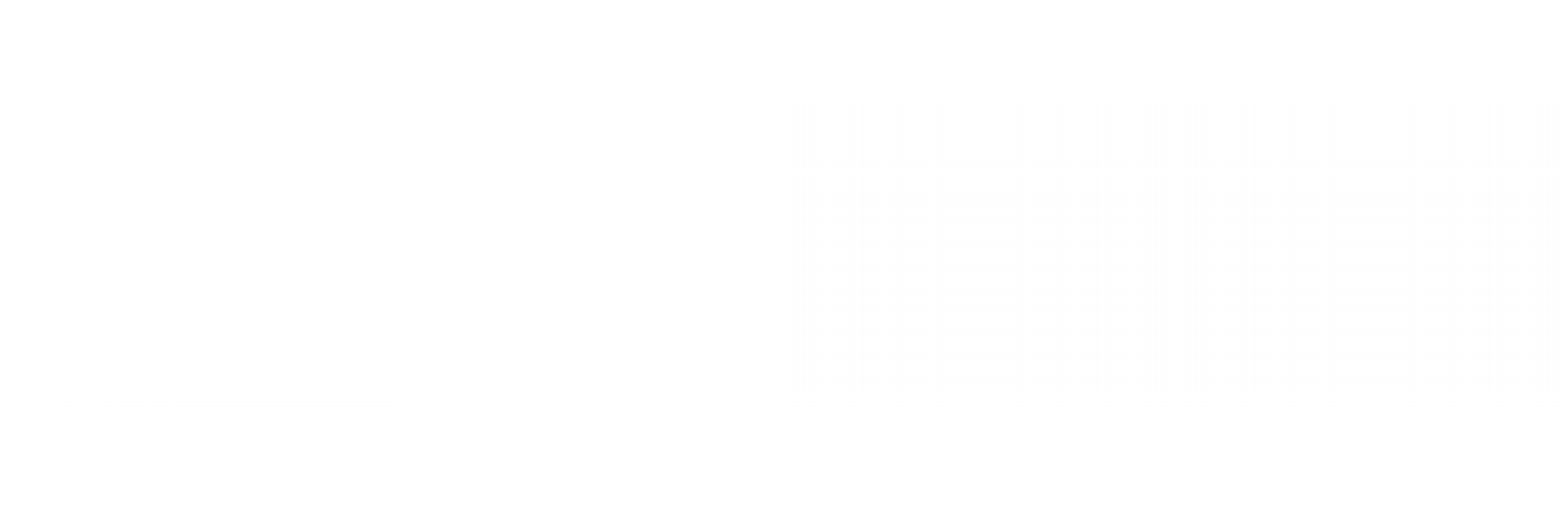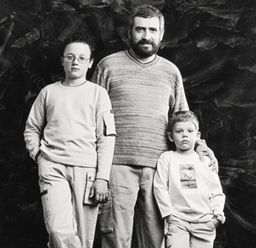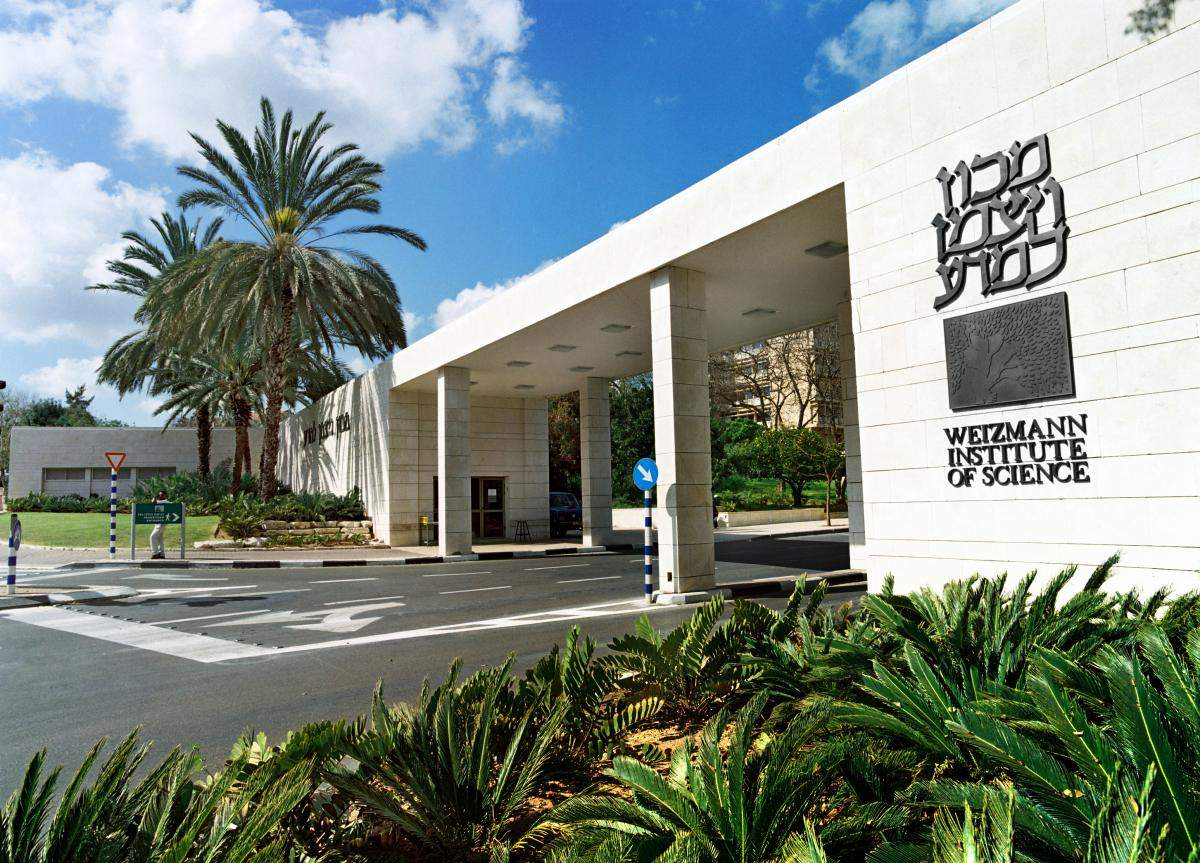# A Century-Old Challenge

## You are here

### Tags

In 1901 the German mathematician David Hilbert presented a list of twenty-two open problems that he thought would shape the future of mathematics in the 20th century. And indeed, most of Hilbert's problems were solved by the end of the century and in turn gave rise to new branches of mathematical research. However, two of his problems remain unsolved to this day. One of them (no. 16 on Hilbert's list) is the research focus of Prof. Sergei Yakovenko of the Weizmann Institute's Mathematics Department.

The problem can be easily visualized if we take a writing pad and roll it into a tube-like form. Most of the sheets will touch the margins of other sheets, so that a kind of spiral is formed. However, a few sheets may meet their own margins, forming perfect tubes.How many such "tubes" may form when a pad of limitless size is folded? We know that in systems which are described using a square differential equation there can be four "tubes." But what will happen in systems described using differential equations of a higher order (equations raised to higher powers)? Is there a connection between the order of the equation (the power to which it is raised) and the number of "tubes" that may be formed in the system? Having provided a partial solution to these basic questions, Yakovenko's current research efforts aim to completely solve this century-old problem.

### Tags: